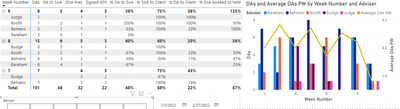cancel
Showing results for
Did you mean:Frequent Visitor

## YTD and Rolling average

HI,

Newly learning my way around so hope someone can help! I am trying to calculate the YTD average of meetings held per week and also show thew rolling average in a bar/line chart visual.

Ive used the following code but am running into issues as the average is being calculated across the numbers of staff each week as opposed to the total for the week.  for example week 9 in the table is being calculated as an average of 2. it's also giving unexpected results in the chart for the same reason, if I filter it to just one team member then it is giving an expected result.The measure I am using is;

Average DAs PW = AVERAGEX(FILTER(ALLSELECTED(Append1),Append1[Week Number]<=MAX(Append1[Week Number])),Append1[DAs])

cheers for any help!

2 REPLIES 2Super User

@Phil_SPR , Please create week/Date dimension table and join it with your table on week/date and then try a measure like

Average DAs PW = calculate(AVERAGE(Append1[DAs]), FILTER(ALLSELECTED(Week),Week[Week Number]<=MAX(Week[Week Number])))Frequent Visitor

Thanks for the response, unfortunatly this is giving me the same result.

The issue is that there are anywhere from 2 to 6 advisers taking DAs in any given week and the formula is dividing the weekly DA total between the advisors. Not giving a weekly average of the total.